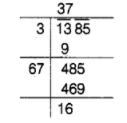# What is the least number that should

Question:

What is the least number that should be added to 6200 to make it a perfect square?

Solution:

First, find the square root of 1385 by long division method.Hence, the least number is 16 , which should be subtracted from 1385 to get a perfect square and the required perfect square number $=1385-16=1369$

$\therefore$ Square root of $1369=\sqrt{37 \times 37}=37$